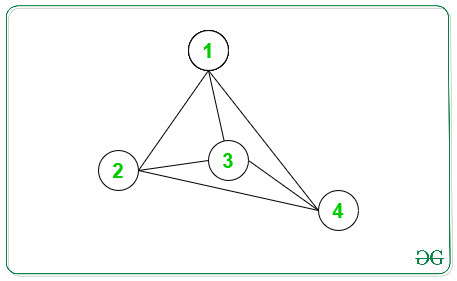Related Articles

# Difference Between sum of degrees of odd and even degree nodes in an Undirected Graph

• Last Updated : 03 Jun, 2021

Given an undirected graph with N vertices and M edges, the task is to find the absolute difference Between the sum of degrees of odd degree nodes and even degree nodes in an undirected Graph.

Examples:

Input: N = 4, edges[][] = { { 1, 2 }, { 1, 3 }, { 1, 4 }, { 2, 3 }, { 2, 4 }, { 3, 4 } }
Output: 12
Explanation:
Below is the graph for the above information:Node -> Degree
1 -> 3
2 -> 3
3 -> 3
4 -> 3
Sum of odd degree node = 3 + 3 + 3 + 3 = 12
Sum of even degree node = 0
Difference = 12

Input: N = 5, edges[][] = { { 1, 2 }, { 1, 3 }, { 2, 4 }, { 2, 5 } }
Output: 4

Approach:

1. For each vertex, the degree can be calculated by the length of the Adjacency List of the given graph at the corresponding vertex.
2. Count the sum of degrees of odd degree nodes and even degree nodes and print the difference.

Below is the implementation of the above approach:

## C++

 `// C++ implementation to print the``// Difference Between sum of degrees``// of odd degree nodes and even``// degree nodes.``#include ``using` `namespace` `std;` `// Function to print the difference``// Between sum of degrees of odd``// degree nodes and even degree nodes.``int` `OddEvenDegree(``int` `N, ``int` `M,``                    ``int` `edges[])``{``    ``// To store Adjacency List of``    ``// a Graph``    ``vector<``int``> Adj[N + 1];``    ` `    ``int` `EvenSum = 0;``    ``int` `OddSum = 0;` `    ``// Make Adjacency List``    ``for` `(``int` `i = 0 ; i < M ; i++) {``        ``int` `x = edges[i];``        ``int` `y = edges[i];` `        ``Adj[x].push_back(y);``        ``Adj[y].push_back(x);``    ``}` `    ``// Traverse each vertex``    ``for` `(``int` `i = 1; i <= N; i++) {` `        ``// Find size of Adjacency List``        ``int` `x = Adj[i].size();` `        ``// If length of Adj[i] is``        ``// an odd number, add``        ``// length in OddSum``        ``if` `(x % 2 != 0)``        ``{``            ``OddSum += x;``        ``}``        ``else``        ``{``            ``// If length of Adj[i] is``            ``// an even number, add``            ``// length in EvenSum``            ``EvenSum += x;``        ``}``            ` `    ``}``    ` `    ``return` `abs``(OddSum - EvenSum);``}` `// Driver code``int` `main()``{``    ``// Vertices and Edges``    ``int` `N = 4, M = 6;` `    ``// Edges``    ``int` `edges[M] = { { 1, 2 }, { 1, 3 }, { 1, 4 },``                       ``{ 2, 3 }, { 2, 4 }, { 3, 4 } };` `    ``// Function Call``    ``cout<< OddEvenDegree(N, M, edges);` `    ``return` `0;``}`

## Java

 `// Java implementation to print the``// difference between sum of degrees``// of odd degree nodes and even``// degree nodes.``import` `java.util.*;` `class` `GFG{` `// Function to print the difference``// between sum of degrees of odd``// degree nodes and even degree nodes.``static` `int` `OddEvenDegree(``int` `N, ``int` `M,``                         ``int` `edges[][])``{``    ` `    ``// To store adjacency list``    ``// of a graph``    ``@SuppressWarnings``(``"unchecked"``)``    ``Vector []Adj = ``new` `Vector[N + ``1``];``    ` `    ``for``(``int` `i = ``0``; i < N + ``1``; i++)``    ``{``       ``Adj[i] = ``new` `Vector();``    ``}``    ` `    ``int` `EvenSum = ``0``;``    ``int` `OddSum = ``0``;` `    ``// Make adjacency list``    ``for``(``int` `i = ``0``; i < M; i++)``    ``{``       ``int` `x = edges[i][``0``];``       ``int` `y = edges[i][``1``];``       ` `       ``Adj[x].add(y);``       ``Adj[y].add(x);``    ``}` `    ``// Traverse each vertex``    ``for``(``int` `i = ``1``; i <= N; i++)``    ``{``        ` `       ``// Find size of adjacency list``       ``int` `x = Adj[i].size();``       ` `       ``// If length of Adj[i] is``       ``// an odd number, add``       ``// length in OddSum``       ``if` `(x % ``2` `!= ``0``)``       ``{``           ``OddSum += x;``       ``}``       ``else``       ``{``           ` `           ``// If length of Adj[i] is``           ``// an even number, add``           ``// length in EvenSum``           ``EvenSum += x;``       ``}``    ``}``    ``return` `Math.abs(OddSum - EvenSum);``}` `// Driver code``public` `static` `void` `main(String[] args)``{``    ` `    ``// Vertices and edges``    ``int` `N = ``4``, M = ``6``;` `    ``// Edges``    ``int` `edges[][] = { { ``1``, ``2` `}, { ``1``, ``3` `}, { ``1``, ``4` `},``                      ``{ ``2``, ``3` `}, { ``2``, ``4` `}, { ``3``, ``4` `} };` `    ``// Function call``    ``System.out.print(OddEvenDegree(N, M, edges));``}``}` `// This code is contributed by PrinciRaj1992`

## Python3

 `# Python3 implementation to print the``# Difference Between sum of degrees``# of odd degree nodes and even``# degree nodes.` `# Function to print the difference``# Between sum of degrees of odd``# degree nodes and even degree nodes.``def` `OddEvenDegree(N, M, edges):` `    ``# To store Adjacency``    ``# List of a Graph``    ``Adj ``=` `[[] ``for` `i ``in` `range``(N ``+` `1``)]``     ` `    ``EvenSum ``=` `0``;``    ``OddSum ``=` `0``;`` ` `    ``# Make Adjacency List``    ``for` `i ``in` `range``(M):``        ``x ``=` `edges[i][``0``];``        ``y ``=` `edges[i][``1``];`` ` `        ``Adj[x].append(y);``        ``Adj[y].append(x);`` ` `    ``# Traverse each vertex``    ``for` `i ``in` `range``(``1``, N ``+` `1``):`` ` `        ``# Find size of``        ``# Adjacency List``        ``x ``=` `len``(Adj[i])`` ` `        ``# If length of Adj[i] is``        ``# an odd number, add``        ``# length in OddSum``        ``if` `(x ``%` `2` `!``=` `0``):``            ``OddSum ``+``=` `x;       ``        ``else``:``            ` `            ``# If length of Adj[i] is``            ``# an even number, add``            ``# length in EvenSum``            ``EvenSum ``+``=` `x;       ``     ` `    ``return` `abs``(OddSum ``-` `EvenSum);` `# Driver code``if` `__name__ ``=``=` `"__main__"``:``    ` `    ``# Vertices and Edges``    ``N ``=` `4``    ``M ``=` `6`` ` `    ``# Edges``    ``edges ``=` `[[``1``, ``2``], [``1``, ``3``],``             ``[``1``, ``4``], [``2``, ``3``],``             ``[``2``, ``4``], [``3``, ``4``]]`` ` `    ``# Function Call``    ``print``(OddEvenDegree(N, M,``                        ``edges));` `# This code is contributed by rutvik_56`

## C#

 `// C# implementation to print the``// difference between sum of degrees``// of odd degree nodes and even``// degree nodes.``using` `System;``using` `System.Collections.Generic;``class` `GFG{` `// Function to print the difference``// between sum of degrees of odd``// degree nodes and even degree nodes.``static` `int` `OddEvenDegree(``int` `N, ``int` `M,``                         ``int` `[,]edges)``{``  ``// To store adjacency list``  ``// of a graph``  ``List<``int``> []Adj = ``new` `List<``int``>[N + 1];` `  ``for``(``int` `i = 0; i < N + 1; i++)``  ``{``    ``Adj[i] = ``new` `List<``int``>();``  ``}` `  ``int` `EvenSum = 0;``  ``int` `OddSum = 0;` `  ``// Make adjacency list``  ``for``(``int` `i = 0; i < M; i++)``  ``{``    ``int` `x = edges[i, 0];``    ``int` `y = edges[i, 1];` `    ``Adj[x].Add(y);``    ``Adj[y].Add(x);``  ``}` `  ``// Traverse each vertex``  ``for``(``int` `i = 1; i <= N; i++)``  ``{``    ``// Find size of adjacency list``    ``int` `x = Adj[i].Count;` `    ``// If length of Adj[i] is``    ``// an odd number, add``    ``// length in OddSum``    ``if` `(x % 2 != 0)``    ``{``      ``OddSum += x;``    ``}``    ``else``    ``{``      ``// If length of Adj[i] is``      ``// an even number, add``      ``// length in EvenSum``      ``EvenSum += x;``    ``}``  ``}``  ``return` `Math.Abs(OddSum - EvenSum);``}` `// Driver code``public` `static` `void` `Main(String[] args)``{``  ``// Vertices and edges``  ``int` `N = 4, M = 6;` `  ``// Edges``  ``int` `[,]edges = {{1, 2}, {1, 3}, {1, 4},``                  ``{2, 3}, {2, 4}, {3, 4}};` `  ``// Function call``  ``Console.Write(OddEvenDegree(N, M, edges));``}``}` `// This code is contributed by Princi Singh`

## Javascript

 ``
Output:
`12`My Personal Notes arrow_drop_up